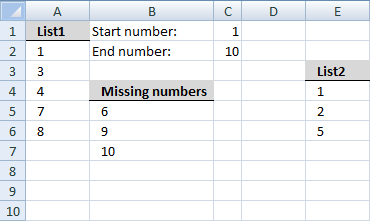Author: Oscar Cronquist Article last updated on August 12, 2018

### Question:

I want to find missing numbers in two ranges combined? They are not adjacent.### Array formula in cell B5:

=SMALL(IF((COUNTIF(List1, ROW(INDEX(\$A:\$A, \$F\$2):INDEX(\$A:\$A, \$F\$3)))+COUNTIF(List2, ROW(INDEX(\$A:\$A, \$F\$2):INDEX(\$A:\$A, \$F\$3))))=0, ROW(INDEX(\$A:\$A, \$F\$2):INDEX(\$A:\$A, \$F\$3)), ""), ROW(A1)))

How to create an array formula

1. Select cell B5
2. Press with left mouse button on in formula bar3. Copy and paste array formula to formula bar
4. Press and hold Ctrl + Shift
5. Press Enter
6. Release all keys

### To remove #NUM errors, Excel 2007 users can use this formula:

=IFERROR(SMALL(IF((COUNTIF(List1, ROW(INDEX(\$A:\$A, \$F\$2):INDEX(\$A:\$A, \$F\$3)))+COUNTIF(List2, ROW(INDEX(\$A:\$A, \$F\$2):INDEX(\$A:\$A, \$F\$3))))=0, ROW(INDEX(\$A:\$A, \$F\$2):INDEX(\$A:\$A, \$F\$3)), ""), ROW(A1)), "")

### Named ranges

List1 (A2:A6)
List2 (E4:E6)
missing_list_start (B5)
What is named ranges?

### Explaining array formula in cell B5

=SMALL(IF((COUNTIF(List1, ROW(INDEX(\$A:\$A, \$F\$2):INDEX(\$A:\$A, \$F\$3)))+COUNTIF(List2, ROW(INDEX(\$A:\$A, \$F\$2):INDEX(\$A:\$A, \$F\$3))))=0, ROW(INDEX(\$A:\$A, \$F\$2):INDEX(\$A:\$A, \$F\$3)), ""), ROW(A1)))

Step 1 - Create dynamic array with numbers from start to end

=SMALL(IF((COUNTIF(List1, ROW(INDEX(\$A:\$A, \$F\$2):INDEX(\$A:\$A, \$F\$3)))+COUNTIF(List2, ROW(INDEX(\$A:\$A, \$F\$2):INDEX(\$A:\$A, \$F\$3))))=0, ROW(INDEX(\$A:\$A, \$F\$2):INDEX(\$A:\$A, \$F\$3)), ""), ROW(A1)))

ROW(INDEX(\$A:\$A, \$F\$2):INDEX(\$A:\$A, \$F\$3))

becomes

ROW(INDEX(\$A:\$A, 1):INDEX(\$A:\$A, 10))

becomes

ROW(\$A\$1:INDEX(\$A:\$A, 10))

becomes

ROW(\$A\$1:\$A\$10)

and returns this array:

{1; 2; 3; 4; 5; 6; 7; 8; 9; 10}

Step 2 - Find missing numbers

=SMALL(IF((COUNTIF(List1, ROW(INDEX(\$A:\$A, \$F\$2):INDEX(\$A:\$A, \$F\$3)))+COUNTIF(List2, ROW(INDEX(\$A:\$A, \$F\$2):INDEX(\$A:\$A, \$F\$3))))=0, ROW(INDEX(\$A:\$A, \$F\$2):INDEX(\$A:\$A, \$F\$3)), ""), ROW(A1)))

(COUNTIF(List1, ROW(INDEX(\$A:\$A, \$F\$2):INDEX(\$A:\$A, \$F\$3)))+COUNTIF(List2, ROW(INDEX(\$A:\$A, \$F\$2):INDEX(\$A:\$A, \$F\$3))))

becomes

(COUNTIF(List1, {1; 2; 3; 4; 5; 6; 7; 8; 9; 10})+COUNTIF(List2, {1; 2; 3; 4; 5; 6; 7; 8; 9; 10}))

becomes

(COUNTIF({1;3;4;7;8}, {1; 2; 3; 4; 5; 6; 7; 8; 9; 10})+COUNTIF({1;2;5}, {1; 2; 3; 4; 5; 6; 7; 8; 9; 10}))

{0;1;1;0;0;1;1;0;0} + {1;0;0;1;0;0;0;0;0}

and returns

{1;1;1;1;0;1;1;0;0}

Step 3 - Convert boolean array into missing numbers

=SMALL(IF((COUNTIF(List1, ROW(INDEX(\$A:\$A, \$F\$2):INDEX(\$A:\$A, \$F\$3)))+COUNTIF(List2, ROW(INDEX(\$A:\$A, \$F\$2):INDEX(\$A:\$A, \$F\$3))))=0, ROW(INDEX(\$A:\$A, \$F\$2):INDEX(\$A:\$A, \$F\$3)), ""), ROW(A1)))

IF((COUNTIF(List1, ROW(INDEX(\$A:\$A, \$F\$2):INDEX(\$A:\$A, \$F\$3)))+COUNTIF(List2, ROW(INDEX(\$A:\$A, \$F\$2):INDEX(\$A:\$A, \$F\$3))))=0, ROW(INDEX(\$A:\$A, \$F\$2):INDEX(\$A:\$A, \$F\$3)), "")

becomes

IF(({1;1;1;1;0;1;1;0;0}, ROW(INDEX(\$A:\$A, \$F\$2):INDEX(\$A:\$A, \$F\$3)), "")

becomes

IF(({1;1;1;1;0;1;1;0;0}, {1; 2; 3; 4; 5; 6; 7; 8; 9; 10}, "")

and returns

{"";"";"";"";6;"";"";9;10}

Step 4 - Return the k-th smallest number

=SMALL(IF((COUNTIF(List1, ROW(INDEX(\$A:\$A, \$F\$2):INDEX(\$A:\$A, \$F\$3)))+COUNTIF(List2, ROW(INDEX(\$A:\$A, \$F\$2):INDEX(\$A:\$A, \$F\$3))))=0, ROW(INDEX(\$A:\$A, \$F\$2):INDEX(\$A:\$A, \$F\$3)), ""), ROW(A1)))

becomes

=SMALL({"";"";"";"";6;"";"";9;10}, ROW(A1))

becomes

=SMALL({"";"";"";"";6;"";"";9;10}, 1)

returns the number 6 in cell B5.